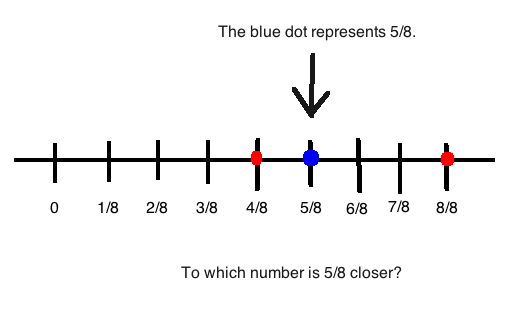# Is 5/8 closer to 1/2 or 1?

To determine if a fraction is closer to one number or the other, make sure all numbers have the same common denominator.

So, let's take 1/2 first. What number do we need to multiply to the denominator so that it equals 8? (Since we're trying to make all the denominators equal to the same number, which is 8 in this case.) You would have to multiply 4 to it to make the denominator 8. But, as a rule of fractions, you have to multiply the numerator by the same number that you multiply the denominator with. So multiply 4 to both the denominator and the numerator.

(1 * 4)/(2 * 4) = 4/8 <-- This is the first number.

Now for the second number, 1, we have to make it have a denominator of 8. Would you agree with me if I said that 1 = 1/1? With a denominator of 1? Because any number divided by 1 still gives you the same number. Now, what number do you multiply the denominator with to get 8? It would have to be 8. And as a rule of fractions, you have to multiply the numerator by the same number, too.

(1 * 8)/(1 * 8) = 8/8 <-- This is the second number.

Now this makes answering this question easier. Is 5/8 closer to 4/8 or 8/8? Take a look on this number line:You can visually see that the blue dot (5/8) is closer to the red dot on the left (4/8), and 4/8 is equal to 1/2. Therefore, 5/8 is closer to 1/2.

thanked the writer.